Geometric progression

(diff) ← Older revision | Latest revision (diff) | Newer revision → (diff)

A sequence of numbers each one of which is equal to the preceding one multiplied by a number(the denominator of the progression). A geometric progression is called increasing if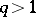, and decreasing if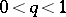; if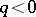, one has a sign-alternating progression. Any term of a geometric progressioncan be expressed by its first termand the denominatorby the formula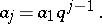The sum of the firstterms of a geometric progression (with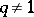) is given by the formulaIf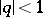, the sum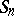tends to the limitasincreases without limit. This numberis known as the sum of the infinitely-decreasing geometric progression.

The expression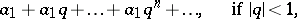is the simplest example of a convergent series — a geometric series; the number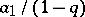is the sum of the geometric series.

The term "geometric progression" is connected with the following property of any term of a geometric progression with positive terms: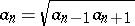, i.e. any term is the geometric mean of the term which precedes it and the term which follows it.

How to Cite This Entry:
Geometric progression. Encyclopedia of Mathematics. URL: http://www.encyclopediaofmath.org/index.php?title=Geometric_progression&oldid=12512
This article was adapted from an original article by O.A. Ivanova (originator), which appeared in Encyclopedia of Mathematics - ISBN 1402006098. See original article